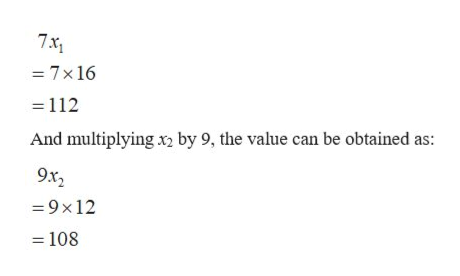# Solve for x1 and x2 2x1 + 4x2 = 803x1 + 1x2 = 60and then when find x1 multiply by 7 and when find x2 multiply by 9 what is the sum of x1 and x2

Question
41 views

Solve for x1 and x2

2x1 + 4x2 = 80

3x1 + 1x2 = 60

and then when find x1 multiply by 7

and when find x2 multiply by 9

what is the sum of x1 and x2

check_circle

Step 1

Consider the equation,

2x1+4x2=80……… (1)

3x1+1x2=60……… (2)

Multiplying by 4 in equation (2) and then subtracting it from equation (1),

2x1+4x2-12x1-4x2 = 80-240

-10x1 = -160

x= -160/-10

x1 = 16

Substituting the value of x1 in equation (1),

Step 2

The values of x1 and x2 are 16 and 12 respectively.

Multiplying x1 by 7, th...help_outlineImage Transcriptionclose7x1 - 7x 16 112 And multiplying x2 by 9, the value can be obtained as: 9x2 9x12 = 108 fullscreen

### Want to see the full answer?

See Solution

#### Want to see this answer and more?

Solutions are written by subject experts who are available 24/7. Questions are typically answered within 1 hour.*

See Solution
*Response times may vary by subject and question.
Tagged in

### Other